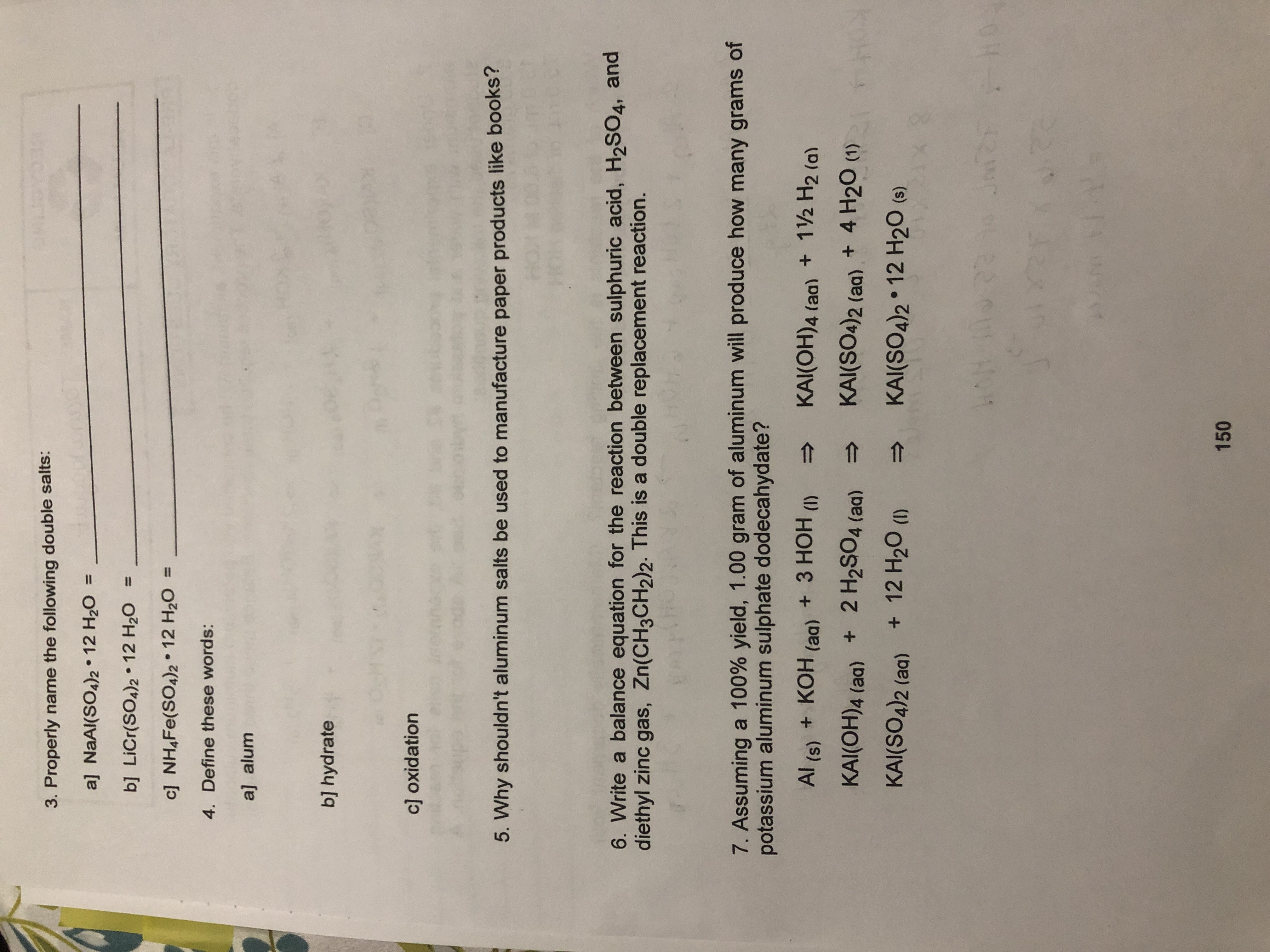# ewktoyot3. Properly name the following double salts:al NaAl(SO4)2 . 12 H20 =bl LiCr(SOA)2 12 H201c] NHAFe(SO4)2 12 H204. Define these words:a] alumbl hydratec] oxidation5. Why shouldn't aluminum salts be used to manufacture paper products like books?.6. Write a balance equation for the reaction between sulphuric acid, H2SO4, anddiethyl zinc gas, Zn(CH3CH2)2. This is a double replacement reaction.7. Assuming a 100% yield, 1.00 gram of aluminum will produce how many grams ofpotassium aluminum sulphate dodecahydate?KAI(OH)4 (aa) + 12 H2 (a)Al (s) + KOH (aq) +3 HOH(1I)KAI(SO4)2 (aq)+ 4 H20 (1)2 H2SO4 (a)KAI(OH)4 (aa)+ 12 H20 ()KAI(SO4)2 (a)KAI(SO4)2 12 H20 (e)1100222N150

Question
9 views

#7help_outlineImage Transcriptioncloseewktoyot 3. Properly name the following double salts: al NaAl(SO4)2 . 12 H20 = bl LiCr(SOA)2 12 H20 1 c] NHAFe(SO4)2 12 H20 4. Define these words: a] alum bl hydrate c] oxidation 5. Why shouldn't aluminum salts be used to manufacture paper products like books? . 6. Write a balance equation for the reaction between sulphuric acid, H2SO4, and diethyl zinc gas, Zn(CH3CH2)2. This is a double replacement reaction. 7. Assuming a 100% yield, 1.00 gram of aluminum will produce how many grams of potassium aluminum sulphate dodecahydate? KAI(OH)4 (aa) + 12 H2 (a) Al (s) + KOH (aq) +3 HOH (1I) KAI(SO4)2 (aq)+ 4 H20 (1) 2 H2SO4 (a) KAI(OH)4 (aa) + 12 H20 () KAI(SO4)2 (a) KAI(SO4)2 12 H20 (e) 110022 2N 150 fullscreen
check_circle

Step 1

Given,

Mass of aluminium = 1.0g

R...

### Want to see the full answer?

See Solution

#### Want to see this answer and more?

Solutions are written by subject experts who are available 24/7. Questions are typically answered within 1 hour.*

See Solution
*Response times may vary by subject and question.
Tagged in

### General Chemistry